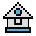Home

# PRESET and PSET Statements

#### Purpose:

To display a point at a specified place on the screen during use of the graphics mode.

#### Syntax:

```PRESET(x,y)[,color]
PSET(x,y)[,color]```

(x,y) represents the coordinates of the point.

color is the color of the point.

Coordinates can be given in either absolute or relative form.

#### Absolute Form

(absolute x, absolute y) is more common and refers directly to a point without regard to the last point referenced. For example:

`(10,10)`

#### Relative Form

STEP (x offset, y offset) is a point relative to the most recent point referenced. For example:

`STEP(10,10)`

Coordinate values can be beyond the edge of the screen. However, values outside the integer range (-32768 to 32767) cause an "Overflow" error.

(0,0) is always the upper-left corner and (0,199) is the lower-left corner in both high resolution and medium resolution.

If the value for color is greater than 3, an "Illegal function call" error is returned.

#### Example 1:

The following draws a diagonal line from (0,0) to (100,100).

```10 CLS
20 SCREEN 1
30 FOR I=0 TO 100
40 PSET (I,I)
50 NEXT
60 LOCATE 14,1```

#### Example 2:

The following clears out the line by setting each pixel to 0.

```40 FOR I=100 TO 0 STEP -1
50 PSET(I,I),0
60 NEXT I```